# Continuous Duty Motor

## Continuous Duty Motor:

There are two types of Continuous Duty Motor — continuous duty at constant load and continuous duty with variable load cycle. In the former the load torque remains constant for a sufficiently longer period corresponding normally to a multiple of time constant of the drive motor. The drive motor is therefore loaded for a sufficient amount of time continuously, till it attains thermal equilibrium.

While driving such a load a motor should have a rating sufficient to drive it without exceeding the specified temperature. The rating of the motor selected for this duty is called its continuous rating or design rating. By Continuous Duty Motor rating one means that it is the maximum load that the motor can give contin­uously over a period of time, without exceeding the temperature rise. Also, the motor selected should be able to withstand momentary overloads. There­fore, the selected motor may sometimes have a rating slightly greater than the power required by the load.

The load diagram and the temperature rise curve of the motor selected for the purpose are shown in Fig. 5.5. Centrifugal pumps, fans, conveyors and compressors are some types of loads where this type of Continuous Duty Motor at constant load is required.Selection of a motor for this class of duty is rather simple and straightfor­ward. From the load characteristics or requirements one can determine the Continuous Duty Motor input required to the mechanical load. A suitable motor may be selected from the catalogue of series manufactured motors. These need not be checked again for thermal or overload capacities. The design rating normally takes care of heating and temperature rise and the motor normally has a short time overload capacity.

While selecting a motor for this type of duty it is not necessary to give importance to the heating caused by losses at starting even though they are more than the losses at rated load. This is because the motor does not require frequent starting. It is started only once in its duty cycle and the losses during starting do not have much influence on heating. However, sometimes it may be necessary to check whether the motor has sufficient starting torque, if the load has considerable amount of inertia.

For most types of loads where the torque and speed are known, the power output of the load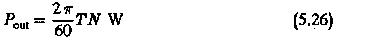If the efficiency of load and transmission is ti the power input to the load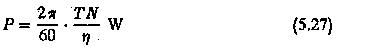In case of linear motion the rating of the motor corresponds to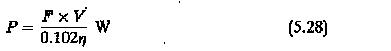where

F is the force exerted by load in kg

V is velocity of motion in m/s

η is efficiency

Using these expressions the rating of the motor is decided. For example the rating of a motor for an elevator is2 in the denominator is by virtue of the counter weight. The rating of the motor for a pump iswhere

ρ density of liquid being pumped.

H head comprising suction,delivery, friction and velocity

Q delivery of pump

η combined efficiency of pump and transmission

The rating of a fan motorwhere

Q volume of air in m3/s.

H pressure of air in mm water or kg/m2

Depending upon the work of a driven machine the rating of the drive motor can be determined.

### Continuous Duty—Variable Load

In this type of duty the load is not constant, but has several steps in one cycle. This cycle of loading repeats for a longer time. If the load variations are slight the motor of Continuous Duty Motor rating of the highest load may be chosen from the available catalog.

However, if the variations in the load cycle are large the machine undergoes a continuous change of temperature. However, after several cycles of operation the motor selected may attain a steady-state value. The thermal calculations of the motor are involved. The selection of a motor based on heating is rather involved and a difficult task. Therefore some simplified criteria may be evolved for selecting a motor for this duty.

If for such a load cycle a motor is selected according to the lowest load, it may not be able to drive the load satisfactorily; the temperature rise of the motor will be exceedingly high and it may not have sufficient capacity to drive the highest load. If the motor is selected according to the highest load, ii becomes overrated and may have poor efficiency. If it is an ac drive motor the pl. is also poor. The motor is underutilized.

The choice of the motor may be based on the average power or average current. At the outset, this method seems to be applicable. It has, however, a disadvantage in that it does not consider the variation of losses. The motor chosen will be smaller for the load cycle, and of insufficient capacity. This may have increased temperature due to overloads, where the losses increase. This happens if the load fluctuations are considerable. The method may give a satisfactory motor if the load fluctuations are relatively small.

However, a method based on average losses of the motor for the load cycle seems to be more appropriate for selecting a motor for a Continuous Duty Motor, variable load. A motor having its rated losses equal to the average of the losses of the motor for the variable load cycle is chosen to drive the load. In this case the final steady-state temperature rise of the motor under variable load is the same as the temperature rise of the motor with constant load. The motor therefore operates with permissible temperature rise.

The selection of a motor based on average losses requires an iterative pro­cedure. A motor whose losses at its rated load are equal to or somewhat greater than the average losses is suitable for the job. However, it may be ex­pected that the motor will have short time peaks of temperature and these may not be very detrimental to the life of the motor. The method does consider maximum temperature rise of the motor under variable load.

A typical load diagram for Continuous Duty Motor variable load is shown in Fig. 5.6. The following steps are involved in the choice of the motor.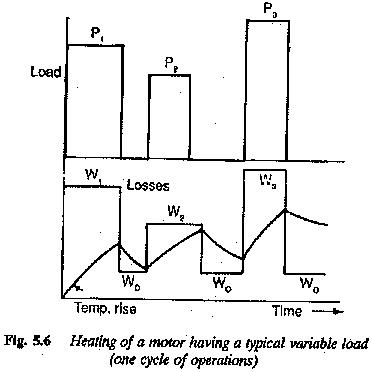1.The average power is determined. The foregoing discussion shows that a motor of this rating is of insufficient capacity. Therefore, a motor is selected from the catalogue, which has a rating 15 to 30% greater than the average

2.For the loads of the load cycle the loss diagram is determined using the efficiency curve of the motor.

3.The average losses are determined using the formula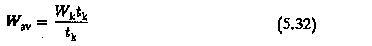4.These losses are compared to the rated losses of the motor (Wr). If Wr is equal to or somewhat greater than Wav, the motor selected is sufficiently good. If Wr is very much greater or less than Wav, the calculations are repeated for a new motor until a right motor is obtained.

5.A check on the overload capacity of the motor must be made. If the motor chosen does not satisfy the overload requirement as per the load cycle, a motor of higher capacity having the overload requirement may be chosen. The basis of heating is disregarded. The motor will however have the thermal rating.

Sometimes it is more convenient to base the selection of motor on equi­valent current, torque or power. These equivalent values are the rms values.

In the equivalent current method, a motor having a rated current equal to the rms value of the variable current of the load cycle is chosen. Let us assume that Ieq is the current rating of such motor. The losses of the motor at rated load are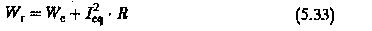When the motor operates on the given load cycle having corresponding current load diagram as shown in Fig 5.7, the losses occurring in the motor with variable load arewhich after simplification is given by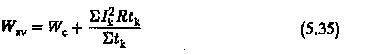Equating these average losses of the motor to its rated losses and simplifying we get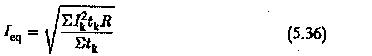Sometimes the load cycle may have a rest period. If the rest period is very large, Ieq determined in the above is on the higher side. Therefore this must be properly taken care of in the calculation. A load diagram with rest period is as shown in Fig. 5.8. The equivalent current for this case is obtained using the relation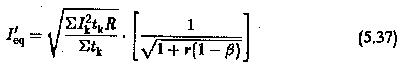where r is the loss ratio Wc/Wr and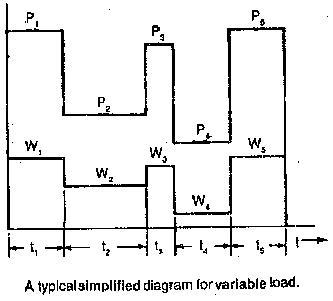If there is a free running period in the cycle, the equivalent current can be calculated using the relation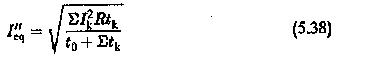Where to is the free running period. It can be seen that the motor chosen will have a rating which lies between the two cases discussed above.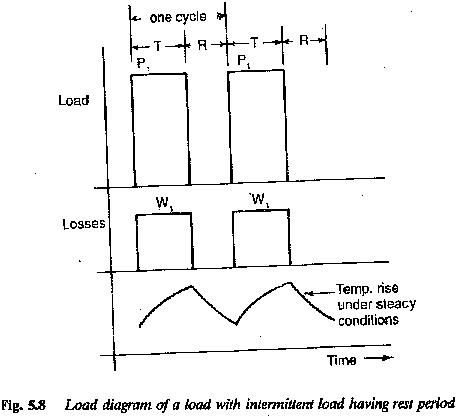Sometimes the loads during intervals of the cycle may not be constant mined using integration. ∑I2ktk in the above equations is replaced by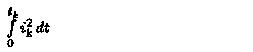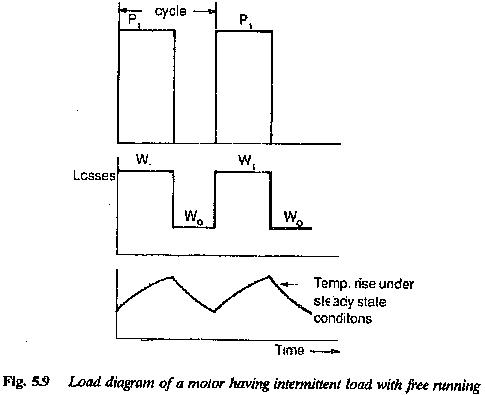If the load diagram shows some arbitrary variation of load during intervals, as shown in Fig. 5.11, some kind of approximation may be made to obtain Equivalent current. The diagram may be approximated by rectangular figures. The calculation will be more accurate if i2 diagram can be drawn before applying the approximation.

After determining the equivalent current, a motor is selected whose rated current is equal to, or slightly greater than, this value.

The motor must be checked for its overload capacity, i.e., one should check that the maximum load in the cycle is less than or equal to permissible overload of the motor. Therefore a chosen motor should have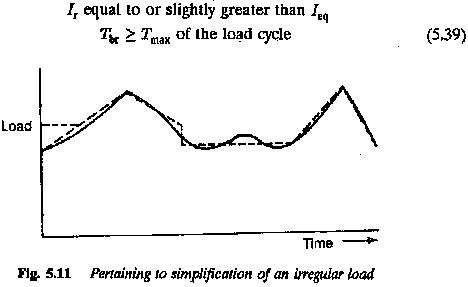If the second condition is not satisfied the motor would be insufficient to drive the load. In this case a motor of higher capacity may be selected according to overload capacity required as per the second condition. The basis of heating need not be considered and the machine will be underutilised as far as the thermal capacity is concerned.

The method of equivalent current gives a right selection if the speed of the motor is constant over the operating cycle. If the speed changes, at lower speeds the cooling will be insufficient in self Cooled machines and the motor may prove thermally ineffective to do the job. The speed variations may have to be taken into consideration in the selection of the motor. If, however, forced ventilation is used, the correction for speed variation may not be necessary. This method is also not applicable to motors having a variation in losses due to skin effect or any other reason. The losses of such motors vary sharply during starting and braking. The method cannot be applied if the ‘constancy’ of so-called constant losses is in question.

When the selection of the motor is based on the equivalent torque criterion, a motor is chosen whose rated torque is equal to (referring to Fig. 5.12).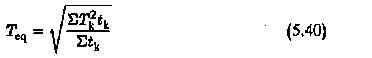If we can assume that the shaft torque is equal to the electromagnetic torque assumption is not true due to friction torque or any other loss torque, the motor may be chosen to have its rated torque greater than Teq accordingly. The selection of the motor under this criterion also assumes constant flux conditions in the motor. Under this assumption the torque will be directly proportional to the armature current. Also, tfiis method assumes constancy of power factor at all loads if ac motors are employed. This method cannot be applied to motors having variable flux conditions, e.g., series motors or squirrel cage motors under starting and braking. Proper corrections may be applied to take into consideration the variable flux condition. The method of equivalent torque is not applicable if the equivalent current method does not give the correct result.

The selection of motor may sometimes be based on equivalent power rating (Fig. 5.13). When this is done, the motor rating is equal to or slightly greater than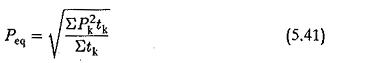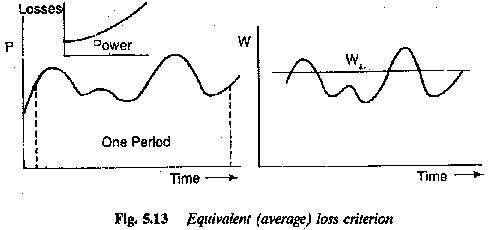The method assumes constant speed at all loads. This method cannot be applied if there are starting and braking periods in the load cycle.

Continuous Duty Motor with variable loading, considered previously, has not taken into account the heating of the motor during starting and stopping. If the starting and braking losses are considerable enough to effect the final temperature rise, they have to be properly considered in the selection of the motor.